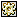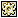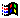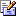Contents Articles Behaviors Books Director News Director Web Sites FAQ Games Mailing Lists News Groups Project Examples Reviews Software Tools Useful Web Sites Utilities Xtras

 Don't miss these#3D Rotation

 Compatibilities:This item has not yet been rated

Author: MatthewGlubb

Rotates a sprite in 3 dimensional space around an axis leading from the mouse pointer to the origin created by Matthew Glubb, May 1997 I'm not to hot on optimisation (works like a dream with 1 bit graphics). I'm sure there"s a more efficient way of doing all those rotation handlers, any takers? Do I *really* need all those floats?

 property x1,y1,z1,whichsprite,xAngle,yAngle,perp1,perp2,whichmember --birth object, set coordinates, initialise settings. on new me,x,y,z,channel   set x1 = x   set y1 = y   set z1 = z   --settings for perspective, change for different effects (try tying these values into mouse location for some wierdness).   set perp1 = 275   set perp2 = 350   set whichsprite = channel   set whichmember = the membernum of sprite whichsprite   puppetsprite whichsprite, TRUE   return me end --call this handler to rotate your sprite. --rotate sprite based on mouse loc (xLoc, yLoc). on hub me,xLoc,yLoc   dorotation me,xLoc,yLoc   workStageCoords me end hub on dorotation me,xLoc,yLoc   set vectorX = xLoc   set vectorY = yLoc   set vectorZ = 100   set xAngle = float(atan(vectorY / vectorZ))   set yAngle = float(atan(vectorX / vectorZ))   rotateXAxis me end dorotation --rotate onto x-axis on rotateXAxis me   set rXy = float(y1 * cos(xAngle)) - (z1 * sin(xAngle))   set rXz = float(y1 * sin(xAngle)) + (z1 * cos(xAngle))   set rXx = x1   rotateYAxis me,rXx,rXy,rXz end rotateXAxis --rotate onto y-axis on rotateYAxis me,rXx,rXy,rXz   set rYz = float(rXz * cos(yAngle)) - (rXx * sin(yAngle))   set rYx = float(rXz * sin(yAngle)) + (rXx * cos(yAngle))   set rYy = rXy   rotateZAxis me,rYx,rYy,rYz end rotateYAxis --rotate around z-axis on rotateZAxis me,rYx,rYy,rYz   --this value controls the rate of rotation.   set angle = 0.075   set rZx = float(rYx * cos(angle)) - (rYy * sin(angle))   set rZy = float(rYx * sin(angle)) + (rYy * cos(angle))   set rZz = rYz   reRotateYAxis me,rZx,rZy,rZz end rotateZAxis --restore to y-axis on reRotateYAxis me,rZx,rZy,rZz   set yAngle = - yAngle   set rYz = float(rZz * cos(yAngle)) - (rZx * sin(yAngle))   set rYx = float(rZz * sin(yAngle)) + (rZx * cos(yAngle))   set rYy = rZy   reRotateXAxis me,rYx,rYy,rYz end rotateYAxis --restore to x-axis on reRotateXAxis me,rYx,rYy,rYz   set xAngle = - xAngle   set y1 = float(rYy * cos(xAngle)) - (rYz * sin(xAngle))   set z1 = float(rYy * sin(xAngle)) + (rYz * cos(xAngle))   set x1 = rYx end rotateXAxis --calculate perspective (height & width) and stage location of sprite. on workStageCoords me   set provX = x1 * (perp1 / (z1 - perp2))   set provY = y1 * (perp1 / (z1 - perp2))   set the locH of sprite whichsprite = provX + ((the stageright - the stageleft) / 2)   set the locV of sprite whichsprite = provY + ((the stagebottom - the stagetop) / 2)                             --experiment with this figure for varying degrees of perspective.                             set the width of sprite whichsprite = the width of member whichmember + (z1 / 8)                             set the height of sprite whichsprite = the height of member whichmember + (z1 / 8) end workStageCoords

 Contact MMI 36 South Court Sq Suite 300 Newnan, GA 30263 USASend e-mail